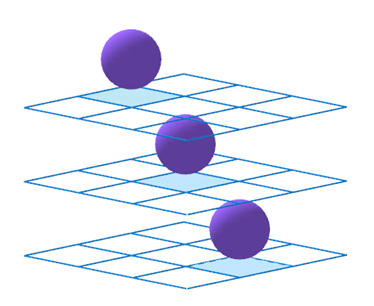#### You may also like### All in the Mind

Imagine you are suspending a cube from one vertex and allowing it to hang freely. What shape does the surface of the water make around the cube?### Three Cubes

Can you work out the dimensions of the three cubes?### Painting Cubes

Imagine you have six different colours of paint. You paint a cube using a different colour for each of the six faces. How many different cubes can be painted using the same set of six colours?

# Marbles in a Box

##### Age 11 to 16Challenge Level

Imagine a three dimensional version of noughts and crosses where two players take it in turn to place different coloured marbles into a box.The box is made from 27 transparent unit cubes arranged in a 3-by-3-by-3 array.

The object of the game is to complete as many winning lines of three marbles as possible.

How many different winning lines are there?

Once you have thought about how you might tackle the problem, click below to see four different methods for working out the number of winning lines in a $3 \times 3 \times 3$ cube.

Alison's method

There are $3$ possible places where a line can start:
• at a vertex
• at the middle of an edge
• in the centre of a face
A cube has $8$ vertices, $12$ edges and $6$ faces.

From a vertex there are $7$ other vertices that you can join to in order to make a winning line.  $7 \times 8 = 56$ lines, but this counts each line from both ends, so there are $28$ 'vertex' winning lines.

From the middle of an edge there are $3$ other middles-of-edges that you can join to in order to make a winning line.  $3 \times 12 = 36$ lines, but this counts each line from both ends so there are $18$ 'middle of edge' winning lines.

From the centre of each face there is one winning line, joining to the opposite face, so there are $3$ 'centre of face' winning lines.

So in total, there are $28 + 18 + 3 = 49$ winning lines.

James' method

Winning lines can either be:
• Diagonal
• Not diagonal
Considering the non-diagonal winning lines first:
There are $9$ from front to back.
There are $9$ from left to right.
There are $9$ from top to bottom.

Considering the diagonal winning lines:

On each layer there are $2$ diagonal winning lines so:
There are $6$ from front to back.
There are $6$ from top to bottom.
There are $6$ from left to right.

There are $4$ lines from a vertex to a diagonally opposite vertex.

In total, there are $27+18+4=49$ winning lines.

Caroline's method

All winning lines must pass either:
• along an edge of the cube
• through the middle of a face
• through the centre of the cube

There are $12$ edges on a cube so there are $12$ winning lines along edges.

There are $6$ faces on a cube, and $4$ winning lines that pass through the middle of each face, so there are $24$ winning lines through the middle of faces.

Finally we need to consider the winning lines that go through the centre cube:

vertex to opposite vertex: $4$
middle of edge to middle of opposite edge: $6$
middle of face to middle of opposite face: $3$

In total, there are $12 + 24 + 4 + 6 + 3 = 49$ winning lines.

Grae's method

The winning lines may be counted by looking at lines:
• in each horizontal plane
• in each vertical plane from left to right
• in each vertical plane from front to back
• in the diagonal planes

On a plane there are $8$ winning lines.
In the cube, there are $3$ horizontal planes, so $8 \times 3 = 24$ winning lines.

There are also $3$ vertical planes going from left to right, but now with only $5$ new winning lines per plane, as the $3$ horizontal lines have already been counted.  So $5 \times 3 = 15$ winning lines.

On the $3$ vertical planes going from front to back, we now only have $2$ new (diagonal) winning lines per plane. So $2 \times 3 = 6$ winning lines.

Finally, there are also diagonal planes to consider. There are $4$ winning lines going from corner to diagonally opposite corner.

In total, there are $24 + 15 + 6 + 4 = 49$ winning lines.

Try to make sense of each method.

Now, try to adapt each method to work out the number of winning lines in a $4 \times 4 \times 4$ cube.

Can you adapt the methods to give a general formula for any size cube?
Check that each method gives you the same formula.

You may be interested in the other problems in our Reasoning Geometrically Feature.